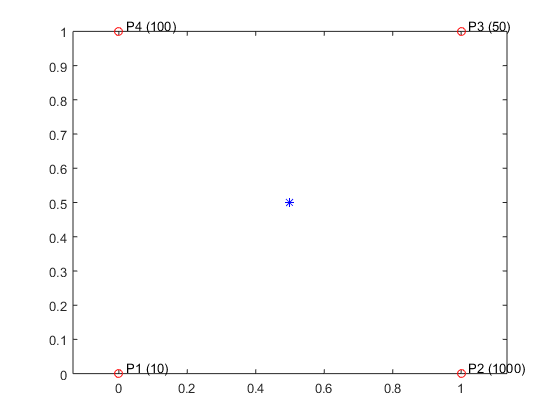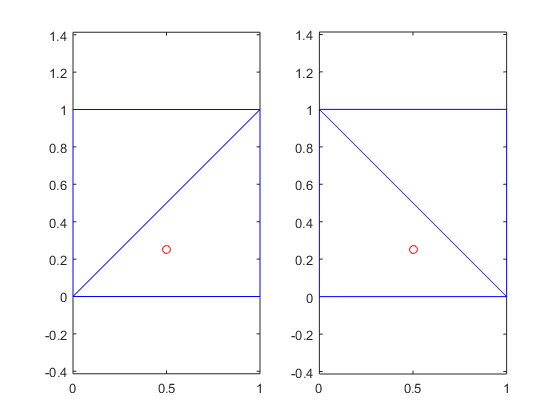# Natural Neighbor – A Superb Interpolation Method

I'm happy to welcome Damian Sheehy as this week's guest blogger. Damian works on the development of geometry-related features at MathWorks. He will provide answers to two frequently asked questions; one on scattered data interpolation that he will cover in this blog and the other on Delaunay triangulation that he will cover in the next. Over to you, Damian...

### Contents

#### An Email from Customer Support

I occasionally get an email from customer support with a title similar to this one: "griddata gives different results. . . ". The support engineers are great, they really know how to choose a good subject line that will get a developer's attention and get a response back to the customer quickly. The subject line could equally well cite scatteredInterpolant as it shares the same underlying code as griddata. Before I open the email I have a strong suspicion about the cause of the difference. If the first line opens with this: "A customer upgraded to MATLAB R20** and griddata gives different results to the previous . . .", then I'm fairly confident what the problem is. I look at the customer's dataset, perform a couple of computations, create a plot, and bingo!, I have a canned response and recommendation at the ready and I turn around the question in a matter of minutes.

#### Why griddata or scatteredInterpolant May Be Inconsistent

So why should griddata or scatteredInterpolant give different answers after upgrading MATLAB? What has MathWorks done to address this problem? Are there issues with scattered data interpolation that users should be aware of? Yes, there are some subtle behaviors associated with the Nearest Neighbor and Linear interpolation methods for scattered data interpolation. These problems present themselves in specific datasets and the effects may show up as numerical differences after a MATLAB upgrade. I will explain the origin of these problems and the options you have as a user to avoid them altogether. I will also highlight what MathWorks has done to address the problems.

First, let's take a look at the behavior and the data that triggers the problem. To demonstrate, I will choose a simple data set where we have four points at the corners of a square. Each sample point has a different value associated with it and our goal is to compute an interpolated value at some query point within the square. Here's a diagram:

Px = [0; 1; 1; 0];
Py = [0; 0; 1; 1];
V = [10; 1000; 50; 100];
plot(Px, Py, 'or')
hold on
text(Px+0.02, Py+0.02, {'P1 (10)', 'P2 (1000)', 'P3 (50)', 'P4 (100)'})
pq = [0.5 0.5];
plot(pq(:,1), pq(:,2), '*b')
hold off
axis equalFirst, let's consider the Nearest Neighbor interpolation method. For any query point within the square, the interpolated value is the value associated with the nearest neighbor. The figure shown above illustrates the configuration and sample values in parenthesis. We can see an ambiguity arises when the query point lies at the center of the square. There are four possible interpolation solutions and based on the definition of the method any of the four values is a valid solution. Ideally, we would like to have the same result, no matter what computer MATLAB is running on and no matter what version.

This type of problem can also arise with the Linear interpolation method. To perform linear interpolation, the scattered dataset is first triangulated using a Delaunay triangulation. The interpolated value at a query point is then derived from the values of the vertices of the triangle that enclose the point. But a Delaunay triangulation of this dataset is not unique, the illustration below shows two valid configurations.

subplot(1,2,1);
Px = [0; 1; 1; 0; 0; 1];
Py = [0; 0; 1; 1; 0; 1];
pq = [0.5 0.25];
plot(Px, Py, '-b')
hold on
plot(pq(:,1), pq(:,2),'or')
hold off
axis equal
subplot(1,2,2);
Px = [0; 0; 1; 1; 0; 1];
Py = [1; 0; 0; 1; 1; 0];
plot(Px, Py, '-b')
hold on
plot(pq(:,1), pq(:,2),'or')
hold off
axis equal#### Example of Inconsistent Behavior in Linear Interpolation

The interpolated result is different in each scenario. The code to demonstrate this is given in the frame below, I have perturbed one data point to flip the diagonal of the triangulation and illustrate the effect.

P = [0 0; 1 0; 1 1; eps (1-eps);];
V = [10; 1000; 50; 100];
pq = [0.5 0.25];

F = scatteredInterpolant(P,V);
linearVq = F(pq)

P = [0 0; 1 0; 1 1; -eps (1+eps);];
F.Points = P;
linearVq = F(pq)

linearVq =
527.5
linearVq =
267.5


#### Why Natural Neighbor Interpolation is Superior

The interpolated value at the query point, linearVq, is sensitive to how the triangulation edge is created in the tiebreak case. This tiebreak is called a degeneracy and the problem arises in Delaunay triangulations when we have 4 or more cocircular points in 2-D or 5 or more cospherical points in 3-D. Now observe the behavior when we choose the Natural Neighbor interpolation method. This method is also based on an underlying Delaunay triangulation, but it produces the same result in the presence of a diagonal edge swap. Here it is:

P = [0 0; 1 0; 1 1; eps (1-eps);];
F.Points = P;
F.Method = 'natural';
naturalVq = F(pq)

P = [0 0; 1 0; 1 1; -eps (1+eps);];
F.Points = P;
naturalVq = F(pq)

naturalVq =
397.5
naturalVq =
397.5


Natural Neighbor is also a smoother interpolating function, so it makes a lot of sense to favor Natural Neighbor over Linear. Well that begs the question: shouldn’t the Natural Neighbor method be the default? This would be our preferred choice of default, but this method was added to MATLAB long after the griddata Linear method was introduced. Changing a default would have a significant impact, so the change would be more disruptive than the problem it addresses. The Natural Neighbor method is also more computationally expensive, so for large datasets Linear may be preferred for performance reasons.

The example we just reviewed highlights the nature of the problem and gives you a more stable alternative to avoid potential differences from scattered data interpolation after you upgrade MATLAB. For our part here in development, we have long recognized these types of issues create problems for users and we have adopted better underlying algorithms to address them. If you are a long-time user of MATLAB and the griddata function, you may recall more annoying past behavior. Prior to R2009a, repeated calls to the function using the now redundant {'QJ'} Qhull option gave potentially different results on each call. That problem was resolved in R2009a along with the introduction of Natural Neighbor interpolation for its stability and superior interpolation properties. Since then, improvements in the underlying triangulation algorithms have led to stable and consistent results across all platforms, first for 2-D and then for 3-D. Unfortunately, introducing these improvements meant a potential change in behavior of the upgrade for specific datasets, but that's like having to break eggs to make cake. Looking ahead, the behavior of Delaunay triangulation based functions should be much more stable. Of course, fundamental changes to the coded algorithm are likely to trigger changes like the ones we've seen, though the scope of the problem has been reduced substantially.

#### You Tell Me!

I haven't received many support escalations questions related to Natural Neighbor interpolation since it shipped in R2009a. I often wonder if this is because it is working well for users or if users may not be using it. The similar naming to Nearest Neighbor interpolation may cause some to misunderstand the method. How about you, have you used Natural Neighbor interpolation and how has it worked out? Let me know here.

Published with MATLAB® R2015a

|

### Comments

To leave a comment, please click here to sign in to your MathWorks Account or create a new one.